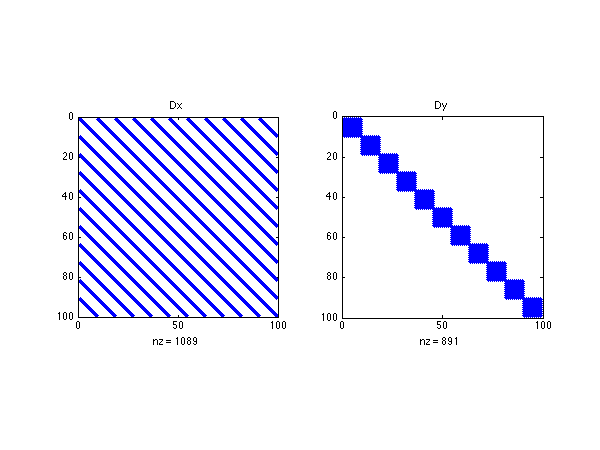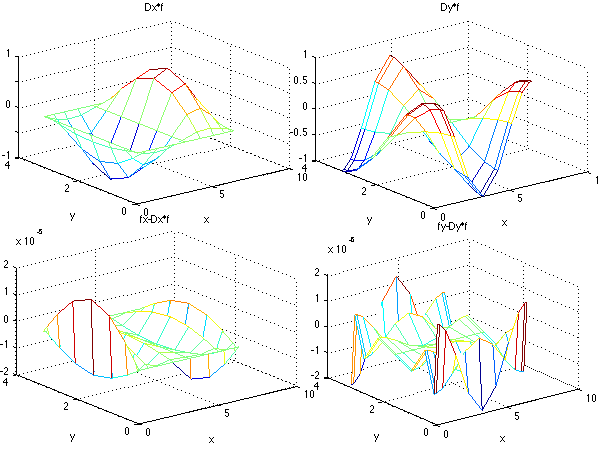# 2D differentiation matrices

This code is just like diffmat_2D.m but here we use dif2D.m to build directly the 2D differentiation matrices. The difference is that here the 1D differentiation matrices are stored as a structure d.x, d.y, d.xx, d.yy, as well as the integration weights d.wx and d.wy. Thus you just need to give d as input argument to dif2D.m.

clear all; clf

%%%% parameters and flags
Nx=11; % gridpoints in x
Ny=9; % gridpoints in x
Lx=2*pi % domain size in x
Ly=pi % domain size in y


# Differentiation matrices

We use the function dif2D.m. The output arguments are

• D: a structure that stores the differenciation matrice for the 2D grid and the integration weights. The structure elements are D.x, D.y, D.xx, D.yy the x and y first and second derivatives differentiation matrices, D.w the integration weights for 2D integration, D.wx the integration weights for integration in x and D.wy the integration weights for integration in y.
• l: the location vectors of indices, used to access the top grid cells l.top, the left grid cells l.left, the right grid cells l.right, the bottom grid cells l.bot. l.cor the indices of the four corners, l.cbl l.ctl l.cbr l.ctr the indices of each individual corner (c for ‘corner’, b for ‘bottom’, l for ‘left’ and r for ‘right’.
• X and Y: the cells location for the 2D grid for x and y, with the structure of the output of the Octave/Matlab function meshgrid.
• Z: a sparse matrix full of zeros whose size is the total number of grid cells.
• I: a sparse identity matrix whose size is the total number of grid cells. Z and I are usefull to build the matrices for the physical systems we study.

And the input arguments are:

• d: the structure comming from the output of dif1D.m containing the 1D grid differentiation matrices and integration weights.
• x and y: the 1D grids in x and y.
% 1D and 2D differentiation matrices
[d.x,d.xx,d.wx,x]=dif1D('cheb',0,Lx,Nx,3);
[d.y,d.yy,d.wy,y]=dif1D('cheb',0,Ly,Ny,3);
[D,l,X,Y,Z,I,NN]=dif2D(d,x,y);


# Test

% Analytical derivatives
f=cos(X).*sin(Y);
fx=-sin(X).*sin(Y);
fy=cos(X).*cos(Y);


# Numerical derivative

% Nuerical derivatives
fX=reshape(D.x*f(:),Ny,Nx);
fY=reshape(D.y*f(:),Ny,Nx);


# Structure of the matrices

Using the spy command we display in a figure the sparsity structure of the two differentiation matrices. We see that they are very different as sketched in the figure above. Since Chebychev differentation matrices in 1D are full, the Dy is block diagonal with dy stacked on the diagonal, whereas Dx is banded.

% showing the structure of D.x and D.y
figure(1)
subplot(1,2,1); spy(D.x); title('D.x');
subplot(1,2,2); spy(D.y); title('D.y');The structure of the matrices

# Comparison

We show the shape of the numerical derivatives and the erreor between the exact derivative and the numerical derivative. We have chosen to take very few gridpoints because otherwise the error would be close to machine accuracy.

% results
figure(2)
subplot(2,2,1);mesh(X,Y,fX); xlabel('x'); ylabel('y'); title('D.x*f');
subplot(2,2,2);mesh(X,Y,fY); xlabel('x'); ylabel('y'); title('D.y*f');
subplot(2,2,3);mesh(X,Y,fx-fX); xlabel('x'); ylabel('y'); title('fx-D.x*f');
subplot(2,2,4);mesh(X,Y,fy-fY); xlabel('x'); ylabel('y'); title('fy-D.y*f');The comparison of exact and numerical derivatives